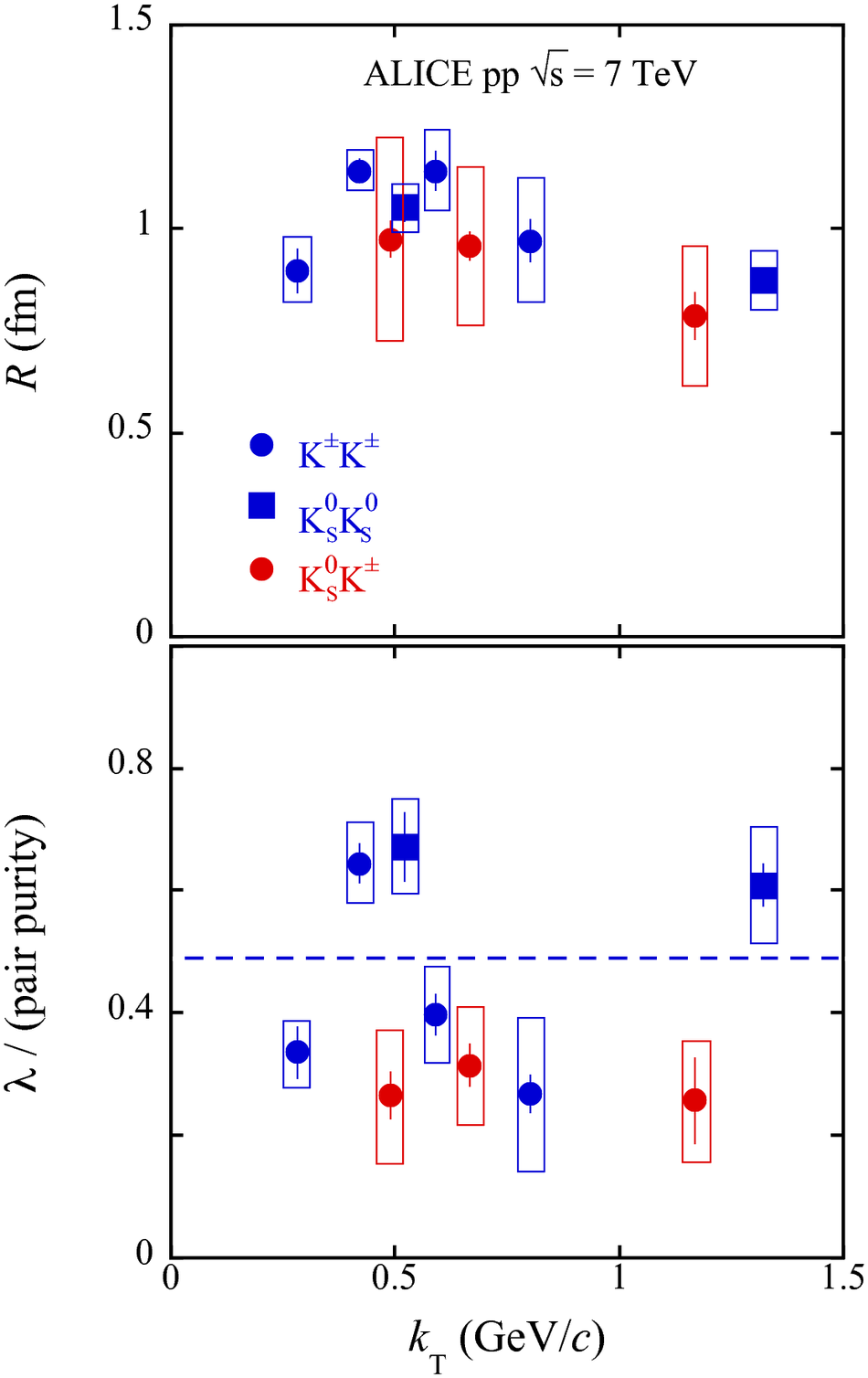# Figure 6

 $R$ and purity-normalized $\lambda$ parameters extracted in the present analysis from K$^0_{\rm S}$K$^{\rm \pm}$ femtoscopy averaged over K$^0_{\rm S}$K$^+$ and K$^0_{\rm S}$K$^-$, along withcomparisons with identical kaon results from ALICE 7 TeV pp collisions averaged overevent multiplicity The quadratic sum of the statistical and systematic uncertainties is plotted for all results as boxes andthe statistical uncertainties are given as lines. Also plotted as a blue dashed lineis the simple average of the identical-kaon purity-normalized $\lambda$ parameters.